# In center

What number lies in the center between a quarter of a fifth and a half of a third on the number axis?

Result

x =  0.108

#### Solution:

$a = \dfrac{ 1 }{ 4 } \cdot \ \dfrac{ 1 }{ 5 } = \dfrac{ 1 }{ 20 } = 0.05 \ \\ b = \dfrac{ 1 }{ 2 } \cdot \ \dfrac{ 1 }{ 3 } = \dfrac{ 1 }{ 6 } \doteq 0.1667 \ \\ \ \\ x = \dfrac{ a+b }{ 2 } = \dfrac{ 0.05+0.1667 }{ 2 } = \dfrac{ 13 }{ 120 } \doteq 0.1083 = 0.108$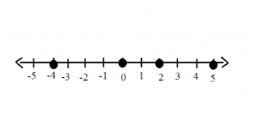Our examples were largely sent or created by pupils and students themselves. Therefore, we would be pleased if you could send us any errors you found, spelling mistakes, or rephasing the example. Thank you!

Leave us a comment of this math problem and its solution (i.e. if it is still somewhat unclear...):Be the first to comment!#### Following knowledge from mathematics are needed to solve this word math problem:

Looking for help with calculating arithmetic mean? Looking for a statistical calculator? Need help calculate sum, simplify or multiply fractions? Try our fraction calculator.

## Next similar math problems:

1. FractionsThree-quarters of an unknown number are 4/5. What is 5/6 of this unknown number?
2. The squareThe square root of 25 times the square root of 81 is what number?
3. Average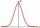Average of 7 numbers is 65. What is its sum?
4. Watching TVOne evening 2/3 students watch TV. Of those students, 3/8 watched a reality show. Of the students that watched the show, 1/4 of them recorded it. What fraction of the students watched and recorded reality tv.
5. Double percent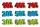What is 80% of 60% of 2800?
6. UN 1If we add to an unknown number his quarter, we get 210. Identify unknown number.
7. AverageThe arithmetic mean of the two numbers is 71.7. One number is 5. Calculate the second number.
8. Expressions with variableThis is algebra. Let n represent an unknown number and write the following expressions: 1. 4 times the sum of 7 and the number x 2. 4 times 7 plus the number x 3. 7 less than the product of 4 and the number x 4. 7 times the quantity 4 more than the nu
9. The ketchup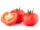If 3 1/4 of tomatoes are needed to make 1 bottle of ketchup. Find the number of tomatoes required to make 4 1/5 bottles
10. Cleaning windowsCleaning company has to wash all the windows of the school. The first day washes one-sixth of the windows of the school, the next day three more windows than the first day and the remaining 18 windows washes on the third day. Calculate how many windows ha
11. Classroom 4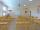In a class of 36 pupils, 2/3 are girls. How much it is in a class girls and boys?
12. Typing course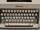Before taking a typing course, Terrence could type 39 words per minute. By the end of the course, he was able to type 68 words per minute. Find the percent increase.
13. Coal mine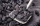The monthly plan of 17,000 tons of coal exceeded the mine by 1/25. How many tonnes of coal have been harvested from the mine above plan?
14. Trees avgThe worker planted 96 trees on Monday, 120 on Tuesday and 61 trees on Wednesday. How many trees did he plant on Thursday if he averaged 105 trees per day?
15. Bag of peanuts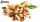Joe eat 1/3 of a bag of peanuts, mark eat 1/4 of the remaining in the bag of peanuts, Alvin eat 1/2 of the remaining bag of peanuts, peter eat 10 peanuts, there are 71 peanuts left. Hon many peanuts were in the bags?
16. InqualitySolve inequality: 3x + 6 > 14
17. Percentages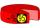Expressed as a percentage: